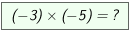Integers ... multiply -2- One positive and one negative number are given. With the sliders factor1 and factor2 you can change the factors. You can let the program show you step by step how to multiply a positive number by a negative one. Click several times on  ? »  If you have understood this, you can solve some problems.